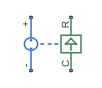# Translational Electromechanical Converter

Interface between electrical and mechanical translational domains

## Library

Electrical Elements

•## Description

The Translational Electromechanical Converter block provides an interface between the electrical and mechanical translational domains. It converts electrical energy into mechanical energy in the form of translational motion, and vice versa. The converter is described with the following equations:

`$F=K·I$`
`$V=K·U$`

where

 `V` Voltage across the electrical ports of the converter `I` Current through the electrical ports of the converter `F` Force `U` Speed `K` Constant of proportionality

The Translational Electromechanical Converter block represents a lossless electromechanical energy conversion, therefore the same constant of proportionality is used in both equations.

Connections + and – are conserving electrical ports corresponding to the positive and negative terminals of the converter, respectively. Connections C and R are conserving mechanical translational ports. If the current flowing from the positive to the negative terminal is positive, then the resulting force is positive acting from port C to port R. This direction can be altered by using a negative value for K.

### Variables

To set the priority and initial target values for the block variables prior to simulation, use the Initial Targets section in the block dialog box or Property Inspector. For more information, see Set Priority and Initial Target for Block Variables.

Nominal values provide a way to specify the expected magnitude of a variable in a model. Using system scaling based on nominal values increases the simulation robustness. Nominal values can come from different sources, one of which is the Nominal Values section in the block dialog box or Property Inspector. For more information, see Modify Nominal Values for a Block Variable.

## Parameters

Constant of proportionality K

Constant of proportionality for electromechanical conversions. The default value is `0.1` V/(m/s).

## Ports

The block has the following ports:

`+`

Electrical conserving port associated with the converter positive terminal.

`-`

Electrical conserving port associated with the converter negative terminal.

`C`

Mechanical translational conserving port.

`R`

Mechanical translational conserving port.

## Version History

Introduced in R2007a